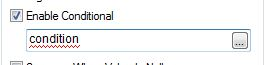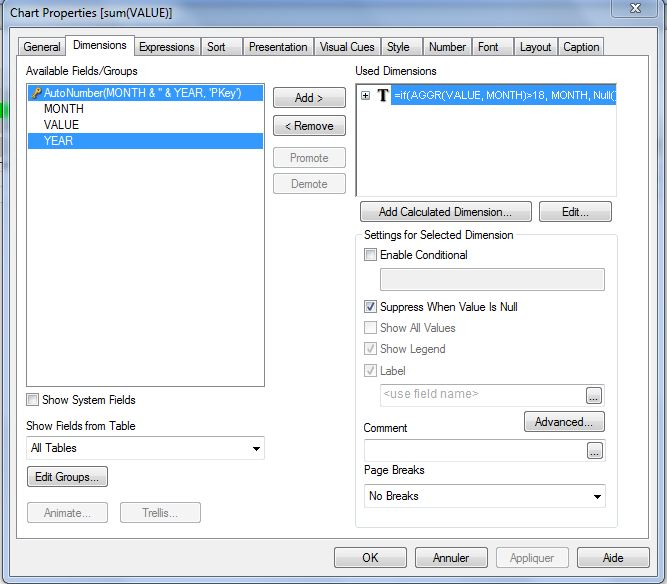# New to QlikView

Discussion board where members can get started with QlikView.

Announcements
The #1 reason QlikView customers adopt Qlik Sense is a desire for a modern BI experience. Read More
cancel
Showing results for
Did you mean:
HighlightedContributor III

## Row condition

Hi all,

i this following table and I would like to show only the rows that met this condition : X>b+2*c

How can I do this ?

Thanks

J

4 Replies
HighlightedNot applicable

If you are only concerned with returning which dimension values meet the above criteria, do the following

In your straight table add the following calculated dimension in the dimension tab

• aggr(if(dimension1>field1+(2*field2),dimension1))
• tick the tickbox that says suppress nulls
• in your expression tab, just add a 1 and then apply

You will be left with a table that shows only dimensions that meet the above criteria. If you want to know exactly what the value was for those met the condition, then instead of 1 in your edit expression, use

• sum(field1)+(2*sum(field2))
HighlightedNot applicable

Hi,

Normally, you should be able to set a condition in the Dimension Tab:Well, it does not work well.

So, Add a calculated dimension and do a conditionnal expression dimensionned by the dimension you want in your report:It should return Null() if the condition is not met, so that you can do "Suppress when value is Null" :Hope this helps

Fabrice

HighlightedPartner

hi you can change your dimension to calculated dimension

and use this formula

aggr(if(X>b+2*c,object1),object1)

HighlightedContributor III

hi

This only works if X>b+2*c are not calc fields

X= sum(K)+ sum(W)

b=avg(Aggr(\$(W),Date)

c=sum(K)/sum(W)

been going insane on this

Plz help